# Inequalities

##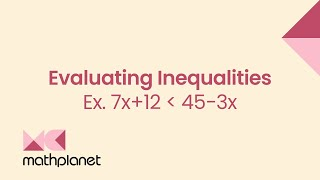By MathPlanetVideos

Determine whether this inequality is true or false for y = 7 y+8<24# Solving inequalities

##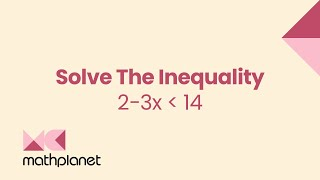By MathPlanetVideos

Solve the inequality 2−3x<14# Solve inequalities

##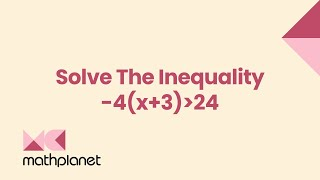By MathPlanetVideos

Solve the inequality -4(x+3)>24# 15.2 Inequalities in a Triangle (Practice)

##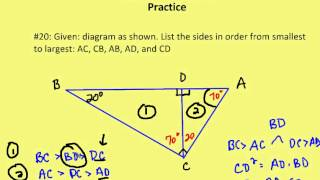By AutenMath

15.2 Inequalities in a Triangle (Practice)# Systems of Linear Inequalities

##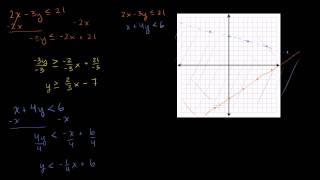By Khan Academy

This Khan Academy video on inequalities offers more information on solving and manipulating systems of inequalities.# Compound inequalities

##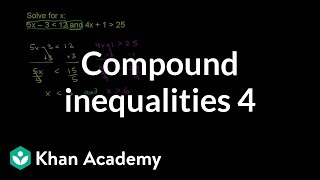By Khan Academy

Sal solves the compound inequality 5x-3<12 AND 4x+1>25, only to realize there's no x-value that makes both inequalities true.# Testing solutions of inequalities

##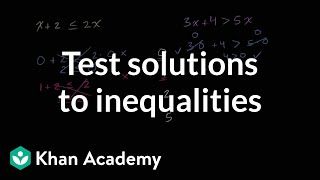By Khan Academy

A solution to an inequality makes that inequality true. Learn how to test if a certain value of a variable makes an inequality true.# Graphing linear inequalities

##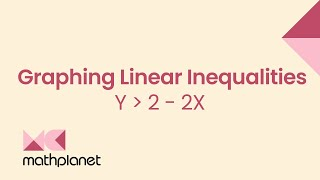By MathPlanetVideos

Graph the inequality y > 2 - 2x# Compound inequalities

##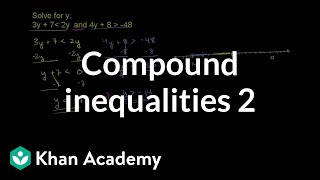By Khan Academy

Sal solves the compound inequality 3y+7<2y AND 4y+8>-48.# One-step inequalities

##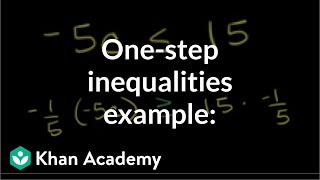By Khan Academy

In addition to solving the inequality, we'll graph the solution. Remember to swap if you mutiply both sides of the inequality by a negative number.# Multi-step linear inequalities

##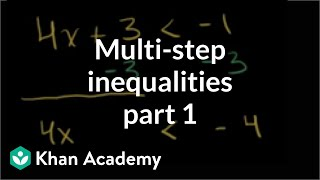By Khan Academy

Sal solves several multi-step linear inequalities.# Graph inequalities and check solutions

##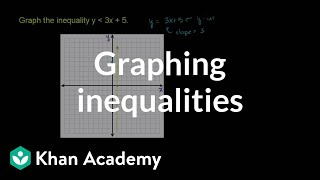By Khan Academy

Sal graphs the inequality y<3x+5.# Graph inequalities and check solutions

##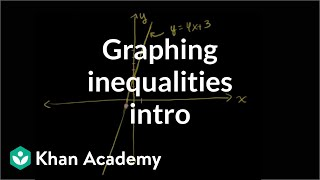By Khan Academy

Learn how to graph two-variable linear inequalities.# 15.2 Inequalities in a Triangle (Lesson)

##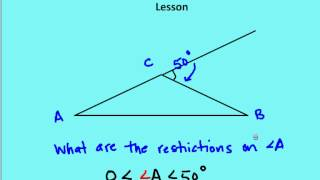By AutenMath

A lesson on the inequality theorems and relationships of side length and angle measures in a triangle# Compound inequalities 2 | Linear inequalities | Algebra I | Khan Academy

##By Khan Academy

Compund Inequalities 2. All Khan Academy content is available for free at www.khanacademy.org# Graphing inequalities 2 | Algebra Basics | Khan Academy

##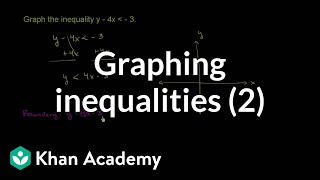By Khan Academy

Watch this example of graphing a strict linear inequality. Notice that the first step is converting the inequality into y < mx + b form.# Multi-step inequalities | Linear inequalities | Algebra I | Khan Academy

##By Khan Academy

In this video, Sal Khan works through some practice problems to explain how to solve multi-step inequalities.# One-step inequalities

##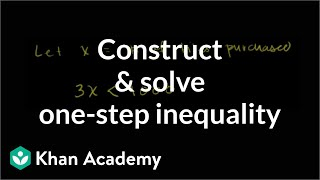By Khan Academy

Inequalities are more than abstract concepts and exercises. They help solve real life problems. Here's an example.# One-step inequalities

##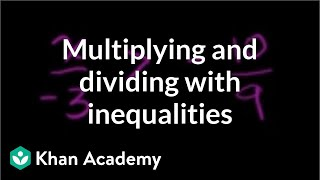By Khan Academy

Our discussion of linear inequalities begins with multiplying and dividing by negative numbers. Listen closely for the word swap. Super important!# Compound inequalities

##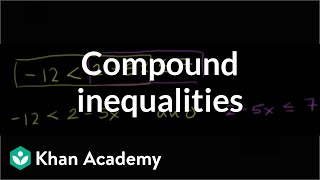By Khan Academy

What happens when you string together more than one inequality? Khan academy lays it down for you.